# Analyzing Performance with Earned Value Analysis

 < Day Day Up >

As discussed in the preceding sections, one way to analyze the status of a project is to look at the cost, work, and finish variances. These measures tell you the impact of experience to date on the total work, total cost, and finish date of the project and its component tasks . One problem with using these variances for analysis is that when one is favorable and another is unfavorable, it's difficult to tell which value is more critical to the project's success. They are measured in different units: the cost variance is measured in dollars, the work variance is measured in hours, and the date variances are measured in time units.

Another problem with using the simple variances is that you can't tell if the costs you have incurred to date have produced as much work and output as you had assumed in the baseline plan. In other words, they don't tell you whether productivity (output per dollar cost) is at the levels you planned. If there is a problem with productivity, then an unfavorable cost variance you might have detected may be just the beginning of an ever-expanding cost variance as work continues on the project and actual values replace estimated values. You can't tell from the simple variances if there is enough left in the budget to finish the project as planned.

Earned value analysis measures performance (productivity) as of a specific date in the life of the projectusually the current date. This allows you to receive early warning signals if a project is not producing the output per dollar cost that you had planned. Earned value analysis makes it possible to compare work and cost variances because work is converted to the dollar value of the work. Therefore, you can compare the value of the work completed to date with the cost of completing it.

NOTE

If the earned value measurements are to accurately reflect performance, your tracking methodology must include not only recording actual start and finish dates, work, and costs, but also rescheduling work not completed on time and work that is completed but that was scheduled after the status date.

#### Understanding Earned Value Measurements

Projects must generate value for their sponsors, or the projects would not be undertaken and funded . Furthermore, that value must be at least as great as the project's estimated cost (its baseline cost) or the sponsors would not approve the project. If the project produces output that is to be sold at a profit, then the buyer must have an even higher value for the project's output. Therefore, you take the baseline cost of the project as a measure of the value of the output of the project. It is at least a minimum measure of the planned value of the project.

When resources perform work on the tasks in a project, they generate the value that the project delivers. Cost accountants like to say that the resources "earn" for the employer the value that will be sold to the buyer. Consequently, the "earned value" of an assignment, a task, or a project at any moment in time is the portion of the planned value that is associated with the work that has been completed.

For example, if you have a project with a baseline cost of \$1 million, then you say that the planned value of the project is \$1 million. The planned value of each summary task, task, and task assignment in this project will also be its baseline cost. If on a specific datesay, the end of Septemberyou find that 60% of all the work that is scheduled for the project has been completed, you say that the earned value as of that date is 60% of the value of the project, or \$600,000. If the baseline schedule called for the project to be 50% complete as of the end of September, then you say that the planned value for that date is \$500,000. Thus, the project appears to be earning value at a faster rate than planned. Finally, suppose that the actual cost as of the end of September is \$550,000. You have spent more than you budgeted to spend by that date! Do you have enough left in the budget to finish the project? There's a high probability that the answer is yes because the value of what has been produced is not only greater than you had planned, but it's also greater than the actual cost.

The productivity is greater than you anticipated, and the project will likely come in at least on time, if not early, and under budget.

The comparison of planned value, earned value, and actual cost helps you assess performance better than simple variances do. Earned value analysis uses these core measurements (planned value, earned value, and actual cost) to create earned value variances and productivity indexes that you can use to predict, based on experience thus far, when the project will finish and what the total cost will be when the project finishes.

Whereas the cost, work, and time variances are based on estimated values at the end of the project, the preceding example makes it clear that earned value analysis compares planned work and cost with actual work and cost as of a given status date . By default, Project uses the current date (which is taken from the computer's system date) as the status date. If you last updated tracking information three days ago but use today's date for the earned value calculations, Project would not have any actual work and cost to offset the planned work and cost for the past three days. Therefore, you generally want to define the status date as the last time you brought actual values up-to-date.

The meanings of the three core earned value measures (planned value, earned value, and actual cost) are summarized as follows :

• Planned value The planned value is the amount of baseline cost that was scheduled to be spent on work up through the status date. PMI formerly called this measure budgeted cost of work scheduled (BCWS) , but it now calls it simply planned value. Microsoft Project 2003 still uses the older term , and the actual field name in Project is BCWS. BCWS is calculated as soon as the baseline is saved, and it is the cumulative sum of baseline costs for each period up to and including the status date.

• Earned value The earned value is that part of planned value that has already been earned by the amount of actual work performed as of the status date. PMI formerly called this the budgeted cost of work performed (BCWP) , but now calls it simply earned value. Project uses both terms, but the actual field name in Project is BCWP.

To calculate earned value, you must determine what portion of a task or assignment has been completed. For tasks, Microsoft Project bases its calculation on the percentage of duration completed (that is, the % Complete field). For assignments, the calculation is based on the percentage of work completed (that is, the % Work Complete field).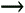See the document "Custom Calculations for Task Earned Value" on the CD accompanying this book for an alternative that calculates earned value for tasks using percent of Work complete.

Note that the percentage complete (however it is measured) is based on the current schedule, not on the baseline amount of duration or work. Suppose a task has a baseline cost of \$1,000 and its baseline work is 100 hours. If actual work as of the status date is 60 hours, but the scheduled work has changed to 120 hours, the task is really only half completed, and only half of its value has been generated thus far. The percentage complete is 50% (60hrs/120hrs), and the earned value is \$500.

If work on a task has been completed as scheduled up to the status date, the earned value and planned value are the same. Differences, however, can arise for several reasons. For example, as just shown, if the scheduled duration and/or work for the task has increased since the baseline was captured, the actual work generates a smaller portion of the total value than was planned. Also, if overtime was used but not scheduled, more work is completed than was scheduled, and earned value is greater than planned value. If a resource has been diverted to another, higher-priority, task or project, the earned valued is less than the planned value.

• Actual cost Actual cost, also called actual cost of work performed (ACWP) , is the actual cost of work that was completed as of the status date. The actual costs of the completed work might differ from the planned costs due to a number of circumstances. For example, you might have actually used overtime that is paid at a premium when none was included in the baseline schedule, resource cost rates might have risen since the baseline was captured, or more expensive resources might have been substituted for those assumed in the original estimates.

NOTE

Cost accrual methods determine when costs are scheduled and when they are recognized as actual costs. Microsoft Project spreads most costs over the duration of the task. The hourly costs of work resources (both standard and overtime costs) and the unit costs of material resources are incurred as work is scheduled for the assignment. By default, fixed cost for a task is also prorated over the duration of the task; but you can change the fixed cost accrual method to Start or End if you want to accrue the total fixed cost at the start or finish of the task. Resource cost per use is always accrued at the start of an assignment. These accrual methods determine when costs show up in the timephased data that is used for variance calculations (and for earned value calculations, as you will see later in this chapter). If the accrual method stipulated for a task's fixed cost is changed after the baseline is captured, that change alone creates a cost variance for status dates between the start and the finish of the task. However, after a task is complete, all costs are counted and the accrual method is no longer an issue.

Microsoft Project calculates the three core earned value measures at the assignment level, the task level, and the summary task level. At the summary task level they are simply the rolled-up sums for subtasks . At the task level, planned value (BCWS) and actual cost (ACWP) are rolled-up sums for the assignments. However, earned value (BCWP) is calculated using a unique formula that only Microsoft Project uses and the result is sometimes different from the rolled-up earned values of the assignments. This formula uses the task % Complete (which is a measure of completed duration), whereas the assignment level calculations use % Work Complete.

The fact that earned value at the task level is not equal to the rolled-up earned values of assignments may not invalidate the use of Microsoft Project's implementation of earned value analysis. The method used is, after all, a weighted average, and it does provide valuable information about performance, even if it's not theoretically accurate. As you will see later, it's often more useful to examine the trend in earned value over time than to calculate a specific number at a moment in time. Because the calculation method is consistent over time, an analysis of the trend is valuable even if you question the precision of an individual calculation.

To illustrate the three core measures and to help you understand what they mean, let's use several variations on a simple example. Assume that Task 1 is scheduled with a duration of 4 days and the resource named Abe is assigned 100% to the task. To keep the math simple, duration is shown in hours (32 hours), and Abe's standard rate is simply \$1. Figure 15.12 illustrates the task and Abe's assignment before any actual work is recorded.

##### Figure 15.12. Project calculates planned value (BCWS) up to the status date as soon as the baseline is captured, but earned value (BCWP) and actual cost (ACWP) require some actual work in order to be calculated.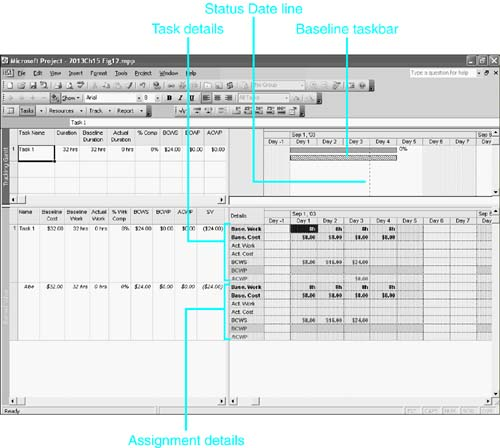Note these features in Figure 15.12:

• The Gantt Chart view in the top pane shows the scheduled taskbar above the baseline taskbar. The dashed vertical line dropping down between Day 3 and Day 4 is the Status Date line; therefore, the status date is Day 3 (September 3), which we will assume is the current date for the project manager.

• The table in the top pane shows the scheduled and baseline duration to be 32 hours, with actual duration and % Complete both 0. The table also shows the task's values for BCWS, BCWP, and ACWP. Because no actual work has been recorded, both BCWP and ACWP are 0.

• The Task Usage view in the bottom pane shows timephased details for Task 1 and for Abe's assignment. The timephased data in the grid shows that baseline work and cost are 8h and \$8 per day. The total baseline cost and work are shown in the table on the left.

• Note that BCWS (planned value) is calculated for each day up through the status date. This happens as soon as the baseline is captured. However, BCWP (earned value) and ACWP (actual value) aren't calculated until actual work is recorded.

• Note that BCWS is a cumulative calculation. Each day's value is that day's baseline cost added to the previous day's BCWS. The BCWS on the status date is the value you see in the table on the left. Earned value (BCWP) and actual cost (ACWP) are also cumulative when calculated, and the value shown on the status date in the grid is the value you see in table's columns on the left.

Now let's assume that it's Day 3 (the status date), and the project manager finds out that Abe has worked only 2 of the 3 days and the task is 50% complete. Figure 15.13 shows what happens to the calculations when the project manager enters 50% in the % Complete field. Project makes actual work equal to scheduled work for each day in the timephased grid until it reaches the 50% point in the task duration. The BCWP and ACWP values for Day 3 are the same as for Day 2 because no additional work was done on Day 3.

##### Figure 15.13. When actual work is recorded, Project calculates the BCWP and ACWP fields up to the status date.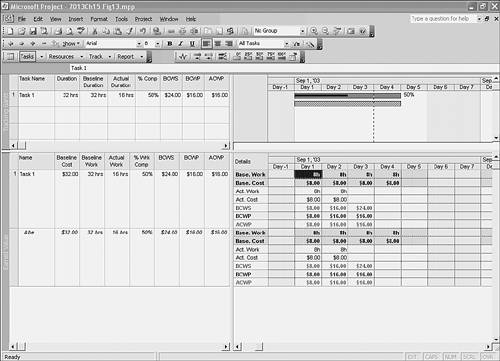Because Abe's assignment is 50% complete, he has earned 50% of the planned value of \$32 (that is, \$16), and the BCWP (earned value) field shows \$16. By comparing earned value with planned value, you see that Abe earned \$8 less value than you had planned, and the reason was because he didn't stay on schedule.

Microsoft Project calculates three earned value schedule indicators that compare earned value with planned value to show you the impact on your project. Figure 15.14 shows the three earned value schedule indicators. They tell you at a glance whether your project is earning value at the rate that was planned. To save space in the figure, all the timephased detail rows except for the earned value fields have been hidden.

##### Figure 15.14. The schedule variance fields SV, SV%, and SPI express the differences between earned value and planned value as a number, a percentage, and a ratio.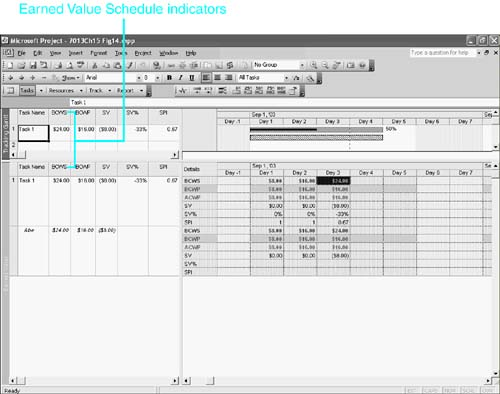The table on the left in both panes in Figure 15.14 is called the Earned Value Schedule Indicators table. This table has all three of the following schedule indicators:

• SV (schedule variance) This field shows the difference between planned value and earned value and is calculated as earned value minus planned value (BCWPBCWS). If SV is negative, as in Figure 15.14, where it's \$8.00, it is unfavorable because it means that the project has earned that much less than it was planned to earn as of the status date. You can think of a negative SV as a shortfall, or deficit, in earned value relative to planned value.

If SV is positive, it is favorable because it means that the project earned more than it had planned to earn as of the status datethe resources did more work than was planned, and the work rate is ahead of the planned work rate. The implication is that the project will likely finish ahead of schedule. You can think of a positive SV as a surplus in earned value relative to planned value.

Because both BCWP and BCWS apply the same budgeted cost to different amounts of work, the difference between them represents a difference between the amount of scheduled work and the amount of actual work. When SV is negative, it means that less work was done on the project than was planned. That's why it's called the schedule variance. Negative values for SV imply that the rate of work is not keeping up with the planned work rate. The implication is that it will be difficult to finish the project on time unless productivity increases or project scope is reduced.

• SV% (schedule variance percentage) This field in Project 2003 expresses SV as a percentage of planned value. Generally, expressing values in percentages is most useful when you're comparing those measurements for tasks or projects that differ substantially in size .

SV% is calculated by dividing SV by BCWS (SV/BCWS). It expresses the earned value deficit or surplus as a percentage of planned value. In this case, where SV% is 33%, it means that the shortfall in earned value was 33% of the planned value. When SV% is positive, it means that there's a surplus in earned value of that percentage. A negative value for SV% implies that something is keeping work from being completed on time, and the project manager should determine what factors are at play in order to avoid missing the finish goals. It might suggest that additional resources should be assigned to the task or project. A positive value implies that work is running ahead of schedule, and it might be possible to reallocate some resources to other tasks or projects and still meet the project's deadline.

Note that SV% is not calculated at the assignment level.

• SPI (schedule performance index) This is the ratio of earned value to planned value (BCWP/BCWS). In our example it is \$0.67 (\$16/\$24), and it means that the project earned only \$0.67 of every \$1 of value that we planned for it to earn. In terms of productivity, it means that the project was only 0.67 as productive as we planned for it to be. Values lower than 1.0 are unfavorable, with the same implications as negative values for SV%. Values over 1.0 are favorable and imply the same things that positive SV% values imply.

The SPI can be used to estimate the project completion date by dividing the remaining duration by the SPI. In our example, half of the task's duration (16 hours) remains to be completed after the status date. If productivity continues at the same rate, it will take approximately 24 more hours (Remaining Duration/SPI, or 16h/0.67) to finish the project. That's 3 more days from the status date (Day 3), and it would put the finish date on Day 6 of the project instead of on Day 4, as scheduled.

Note that SPI is not calculated at the assignment level.

In the preceding example, earned value (BCWP) and actual cost (ACWP) are the same. To illustrate how they might differ, and what that would mean, let's modify the example and assume that although Abe was scheduled to perform the work, he was unavailable at the last minute, and the project manager assigned Sam to replace Abe. Thus, Sam worked only 2 days. However, Sam's standard rate is 25% higher than Abe's, \$1.25 per hour . Obviously, actual costs will be higher than planned.

When the resource substitution is recorded and 50% actual % Complete is recorded, the calculations look like those in Figure 15.15.

##### Figure 15.15. You can compare the BCWP and ACWP fields to see whether you spent more than the planned value of the work that was produced.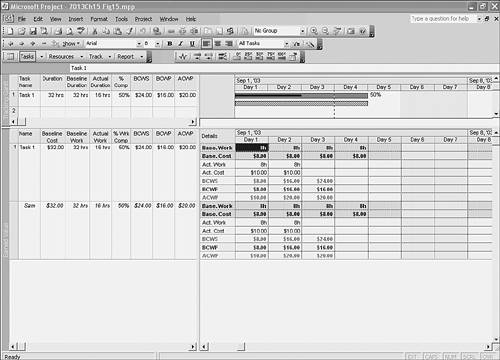The timephased and total values for planned value (BCWS, \$24) and earned value (BCWP, \$16) in Figure 15.15 are the same as those for Abe in Figure 15.13. Consequently, you would have the same SV (schedule variance, \$8). In this example, though, the timephased actual cost is \$10 per day instead of the budgeted (that is, baseline) \$8 per day, and the actual cost (ACWP) as of the status date is \$20. The earned value up through the status date is \$16 (that was the planned value of the actual work), but the actual cost was \$20. Therefore, it cost \$20 to generate the earned value of \$16. The " overrun " of \$4 clearly has negative implications for the final cost of the project if it is a pattern that is repeated.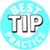Many organizations consider favorable variances to be poor project management. Increased scope encourages gold- plating , a practice of delivering more than expected with little added value to the customer. Underbudget expenses and finishing early imply excessive padding to both time and cost estimates, and are usually scrutinized by a project's sponsor in future projects. In addition, the early release of resources means underutilization because people can rarely start right away on their next assignment. It's like a plane arriving early at an airport: It typically means waiting on the tarmac for the assigned gate to become available or an extended wait for passengers making connections. Experience shows that customers are most appreciative of delivery of what is expectedno more, no less.

A comparison of BCWP and ACWP produces cost indicators similar to the schedule indicators shown in Figure 15.14. Figure 15.16 shows the same example, with the earned value cost variance fields displayed. The table on the left of each view is named the Earned Value Cost Indicators table.

##### Figure 15.16. The Earned Value Cost Indicators table summarizes the effectiveness of cost thus far in generating the planned value.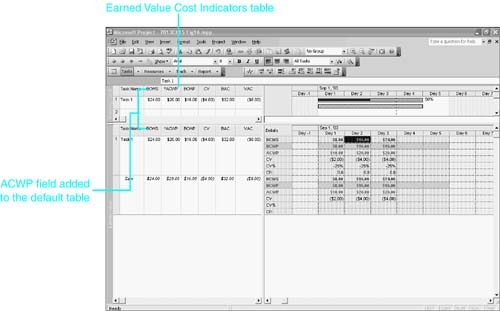NOTE

Note that the Earned Value Cost Indicators table contains the BCWS field but does not, by default, include the ACWP field. Because ACWP is used in calculating the indicators, and BCWS is not, the table in Figure 15.16 has been modified to include the ACWP field. For this illustration it's titled *ACWP, to remind you that it's not by default included in the table.

You should redefine the table and store the redefined table in your Global template. To redefine the table, choose View, Table, More Tables, Earned Value Cost Indicators, and click Edit. Select BCWS in the Field Name column and change it to ACWP. Click OK to save the definition in the active project. Click the Organizer button to display the Organizer dialog box. Select the Earned Value Cost Indicators table in the list on the right (the list of table definitions in the active project). Click the Copy button to copy that definition to the Global template. Click Yes to replace the current definition in the template. Then click Close to close the Organizer and click Close to close the More Tables dialog box.For more information about managing the template, see "Working with the Organizer and the Global File," p. 107 .

The cost indicators in Figure 15.16 have the following meanings:

• CV (cost variance) This indicator shows the difference between earned value and actual cost, and is calculated as earned value minus actual cost (BCWPACWP). In this example the CV is \$4, and it means that the actual cost of production was \$4 more than the earned value of the output. Because BCWP and ACWP both apply cost to the same amount of work, the difference between them represents a difference between the budgeted and actual cost rates. If CV is positive, it is favorable because it means the earned value of the work was greater than the cost to produce it. If CV is negative, it is unfavorable because it means the value of the work was lower than the cost to produce ityou spent the amount of CV over and above the value earned.

• CV% (cost variance percentage) This is a field that expresses CV as a percentage of earned value (BCWP). It's calculated by dividing CV by BCWP (CV/BCWP). In this example, where it is 25%, it means that you spent 25% more of the budget than was planned for the value that was earned. If this continues, you will not be able to finish the task (and perhaps the project) within budget. Or, to put it another way, you will not have enough left in the budget to complete the remaining work.

Note that CV% is not calculated at the assignment level.

• CPI (cost performance index) This is defined as the ratio of earned value to actual cost (BCWP/ACWP) up through the status date. It is the ratio of budgeted to actual costs for the actual work completed as of the status date. It tells how much value you earn for every dollar of actual cost.

In our example the CPI is 0.80 (\$16/\$20), which means that every dollar of actual cost earned only \$0.80 of planned value.

The CPI is often used to predict whether a project will go over budget and by how much. In fact, Project 2003 includes a field for that calculationthe EAC (estimate at completion) field, which is discussed later in this section.

Note that CPI is not calculated at the assignment level.

There are still more fields to the right of CPI in the Earned Value Cost Indicators table. Figure 15.17 shows those additional fields in the top pane:

• BAC (budget at completion) This is actually the Baseline Cost field, shown with the title BAC. BAC is the term that traditional earned value analysis usually gives to baseline cost. This field shows the total budgeted cost of the task (\$32, in this case). It therefore represents the total planned value of the completed task.

• EAC (estimate at completion) In Project 2000 and earlier versions, this field was equivalent to the Cost field (the total estimated or scheduled cost). In Project 2002 and 2003, EAC calculates an estimate of what the total cost will be when the task is completed if the CPI rate of performance, as of the status date, prevails for the remainder of the project. (To the amount of actual cost already incurred, it adds an estimate for completing the task based on the CPI.)

The formula for the EAC is ACWP+(BACBCWP)/CPI.

The following is an explanation of this formula:

1. First, the actual cost thus farACWPis included (\$20, in this case).

2. Next, you estimate the amount of planned value that has yet to be earned: (BACBCWP).

If the BAC is the planned value for the completed task, then BAC minus earned value thus far (BCWP) is the remaining value to be earned or produced. In this example the BAC is \$32 and the earned value (BCWP) is \$16. Therefore, you still have \$16 of value yet to be earned or generated.

3. Divide the value that's yet to be earned by the CPI, to estimate what it will cost to generate that amount of remaining value at the current performance rate for costs.

The CPI tells you what you are currently earning for each dollar of actual cost you spend. Dividing the remaining value to be earned by the amount you earn for each dollar of actual cost tells you the number of actual cost dollars it will take to generate the remaining value. In this example, the remaining value to be earned is \$16, and you only earn \$0.80 for each dollar of actual cost. Dividing \$16 by 0.8 yields \$20 as the number of dollars it will cost to generate \$16 of value.

4. Adding the \$20 that's already spent to the \$20 that's needed to finish the task gives you \$40 for the EAC.

• VAC (variance at completion) VAC is calculated as BACEAC and is the difference between the total baseline cost (BAC) and the estimated total cost (EAC), given the current rate of performance. In this case, that's \$32 minus \$40, or \$8. If VAC is positive, the cost estimate based on experience so far is lower than budgeted cost. If VAC is negative, the current cost run rate is greater than budgeted and the project will likely run over budget.

• TCPI (to complete performance index) TCPI is the ratio (as of the status date) of the value of the work remaining to be done to the budgeted funds remaining to be spent. The formula is (BACBCWP)/(BACACWP). In this formula, (BACBCWP) is the value of the work remaining to be done and (BACACWP) is the budgeted funds remaining to be spent.

The ratio measures the amount of value each remaining dollar in the budget must earn in order to stay within the budget. If TCPI is greater than 1.0, each remaining dollar in the budget must earn more than \$1 in valuethere is more work to be done than there is budget to cover it, and you either need to increase productivity or reduce the work to be done. A value less than 1.0 means you should be able to complete the project without using all the remaining budgetyou have opportunities to increase scope, quality, or profit, or you can choose to save the money in the budget.

##### Figure 15.17. The cost indicators can be used to estimate the total cost of finishing the project, given the current cost performance index.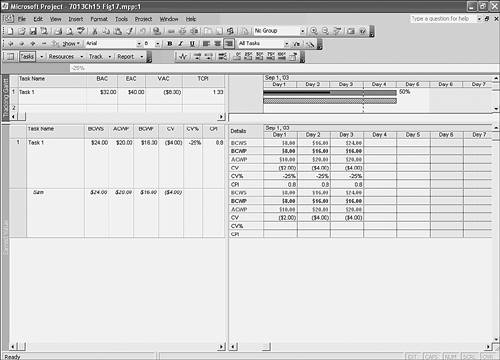#### Controlling the Calculation of Earned Value

Project 2003 provides for an alternative method of calculating earned value (BCWP) at the task level. Sometimes an assignment or a task involves the production of physical units of output or the processing of physical units of input, and you might want to base earned value on the number of units produced (or processed ) as of the status date instead of on the default method, which uses the % Complete field (the percentage of actual duration completed). The Earned Value Method field lets you choose to use Physical % Complete in the calculation instead of % Complete. This field is available at the bottom of the Advanced tab of the Task Information dialog box, where it is labeled Earned Value. It has two possible values: % Complete (which is normally the default) and Physical % Complete. You could also add the field to a table. If you choose to use the Physical % Complete method, you enter that percentage complete to be used in the calculation in the Physical % Complete field, which is now included on the standard Tracking table.

Figure 15.18 shows the Earned Value table with the Earned Value Method and Physical % Complete fields inserted. Tasks 17 and 18 are duplicates of the same task, Assemble First Batch, which is a trial run of a new assembly process. Each version is scheduled for 20 days and is expected to produce 4 fully assembled units. Work has proceeded as scheduled on both versions of the task, and the status date is April 28, which is the 40% complete mark for the task. However, as of the status date, only 1 unit has been assembled . (You assume that the remaining units will go together faster now that the team has worked out the kinks in the process.) The task Assemble First Batch 1 uses the conventional % Complete method of calculating earned value. The task Assemble First Batch 2 uses the Physical % Complete method. Because only 1 of the 4 units is completed, the Physical % Complete is 25%. The baseline cost for each task is \$1,080 (not shown in the figure). The earned value (BCWP) for Assemble First Batch 1 is 40% of \$1,080that is, \$432. The earned value for Assemble First Batch 2 is 25% of \$1,080that is, \$270.

##### Figure 15.18. You can use the Physical % Complete method of calculating earned value for selected tasks.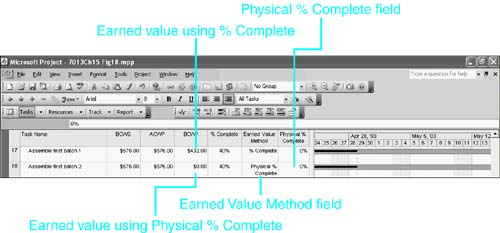Normally the default method of calculation uses % Complete. You can change the default by choosing Tools, Options, to display the Options dialog box. Click the Calculation tab and then click the Earned Value button. The Earned Value dialog box lets you choose the default calculation method (see Figure 15.19). Note that changing the setting affects only tasks that are inserted after the default is changed. If there are existing tasks already in the project, they have the old default value unless you have manually changed them. If you want this to be the default for all your new projects, you can click the Set As Default button. Of course, existing projects are not affected by thistheir default setting will not have been changed, and their tasks will not use the new method. You have to open those projects, change the default calculation method, and change the method for any task that you want to use the new method.

##### Figure 15.19. You can control the calculation of earned value for individual tasks by selecting the method of calculation.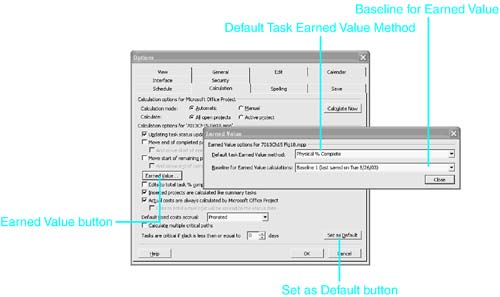Figure 15.19 also reveals another earned value feature in Project 2003. As you learned in Chapter 14, Microsoft Project 2003 allows you to save a total of 11 baselinesthe default baseline plus 10 baselines named Baseline 1 through Baseline 10. In the Earned Value dialog box, the field labeled Baseline for Earned Value lets you choose which of the 11 baselines will be used to calculate earned value. Note that this choice has no effect on the duration, work, cost, start, and finish variancesthey continue to use the standard baseline fields.

For example, if a project schedule had to be revised dramatically after work actually started, you might have decided to capture the revised schedule as Baseline 1. That way you could show progress reports against the original baseline but also show reports against the revised baseline (which might be more meaningful).

#### Using Earned Value Analysis in Project 2003

If you want to view earned value measurements and indicators for a project, you must take three necessary preliminary steps:

1. You must capture the baseline for the project.

2. You must update the project with the latest tracking information. The more thorough your tracking methodology, the more meaningful the earned value indicators will be.

3. You must set the status date to the latest date you entered tracking information.

You can use any of the major task views, but the Gantt Chart view is probably the view that is used most often. There are three tables you can apply to the view to see earned value calculations:

• The Earned Value table This table displays the three core calculations, planned value, earned value, and actual cost (BCWS, BCWP, and ACWP), as well as the earned value schedule and cost variances (SV and CV). It also includes the estimated final cost (EAC), the budgeted cost (BAC), and the variance at completion (VAC). (See Figure 15.20.)

##### Figure 15.20. The Earned Value table shows highlights of the earned value calculations.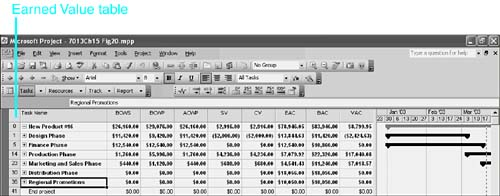• The Earned Value Schedule Indicators table This table focuses on the schedule variance and indicator calculations (refer to Figure 15.14). It includes fields for BCWS, BCWP, SV, SV%, and SPI.

• The Earned Value Cost Indicators table This table focuses on the cost variance and indicator calculations (refer to Figure 15.16). It includes planned value and actual cost, and it includes the earned value cost variance, as CV, CV%, and CPI. It also includes the estimated final cost fields (BAC, EAC, and VAC) and the TCPI field. Recall from the discussion earlier in this chapter that you should permanently modify the table and insert the earned value field (BCWP).

You can display the table you want to use by choosing View, More Views, and then selecting the table you want in the More Views dialog box. Figure 15.20 shows the Earned Value table displayed for the New Product example used for the simple variances earlier in this chapter.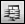Project also provides a custom form that contains the same fields as the Earned Value table (see Figure 15.21). You can display this form to show earned value calculations for a single task or summary task that you have selected. To display the form, display the Custom Forms toolbar (right-click in the toolbar area and choose Custom Forms) and then click Task Earned Value. You can also display the form by choosing Tools, Customize, Forms, and then selecting Task Earned Value in the Customize Forms dialog box.
##### Figure 15.21. The Task Earned Value custom form shows the same fields as the Earned Value table, but for a single task.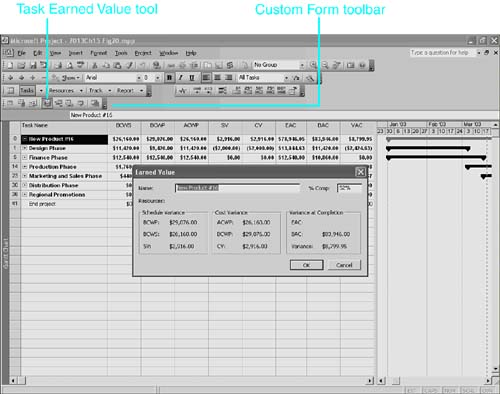#### Creating Earned Value Graphs with Microsoft Excel

As discussed earlier in this chapter, one of the most meaningful ways to analyze earned value data is by examining trends and tendencies. Although hard numbers are informative, they do not provide the whole picture that will best help you manage projects and make informed decisions. Tracking the earned value data graphically over time allows you to see and assess trends in earned value over the life of the project.

Microsoft Project does not generate a graph for earned value, but it can export the timephased data you need to Excel, which can then create the graph for you. The Analyze Timescaled Data in Excel command on the Analysis toolbar exports timephased information into Excel for any of the tasks and fields that you choose. This command lets you export timescaled data for any of the detail rows that you can view in the Task or Resource Usage viewsincluding BCWS, BCWP, and ACWP.

To display the Analysis toolbar, you right-click over a toolbar and choose Analysis from the list of toolbars . When the project you want to analyze is active on the workspace, click the tool named Analyze Timescaled Data in Excel to start the Analyze Timescaled Data Wizard. There are five pages in the wizard. Follow these steps to export the data to Excel:

1. In Step 1 of the Analyze Timescaled Data Wizard (see Figure 15.22), you select the default Entire Project and then click Next. Although you can select specific tasks before starting the wizard and then choose Currently Selected Tasks in this step, to get the totals for the project, you must select Entire Project.

##### Figure 15.22. Choose Entire Project unless you want to graph the data for only a selected set of tasks.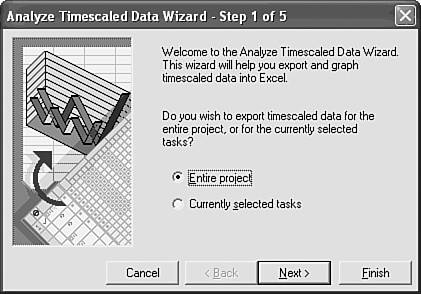2. In Step 2 of the wizard, you select the timephased fields you want to export. For an earned value graph, you can just select BCWS, BCWP, and ACWP. Click each of those fields in the list on the left, and then click the Add button (or you can simply double-click each of the fields). Also, remove the default Work field from the list on the right by clicking it and then clicking Remove (or by simply double-clicking it). Click Next when the list on the right contains BCWS, BCWP, and ACWP (see Figure 15.23).

##### Figure 15.23. In Step 2, you choose the timephased detail fields to be included in the graph.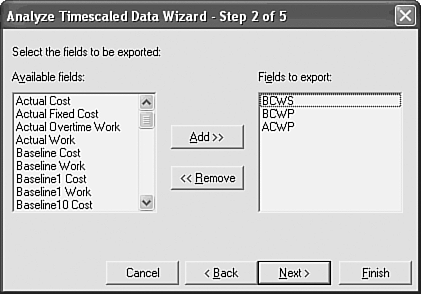3. In Step 3 of the wizard (see Figure 15.24), you define the range of dates you want to include. The project start and finish dates are supplied by default. If you want to concentrate on progress up to the current status date, enter the status date in the To field. Or, if you want to prepare these graphs periodically for comparison purposes, leave the default dates in place. In this example, the default dates for the start and finish of the project have been accepted.

##### Figure 15.24. You should use the date range for the entire project unless you want to focus on a narrower date range.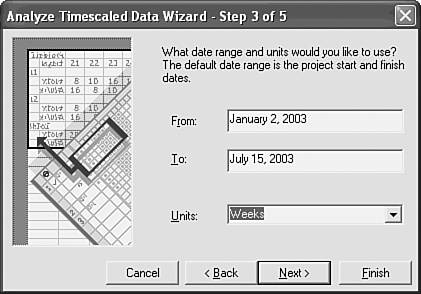In the Units field, select the time period to be plotted in the graph. You can choose Hours, Days, Weeks, Months, Quarters, or Years. This example was created with Weeks as the Units selection.

Click Next after you have chosen the date range and the units.

4. In Step 4 of the wizard (see Figure 15.25), you elect to have Excel generate a graph of the data (by selecting the default Yes, Please) or to export the data only, without creating a graph (by selecting No, Thanks). Click Next after you make a choice. In this example, Yes, Please is selected.

##### Figure 15.25. You can save yourself the time of defining a graph by letting the wizard create one for you.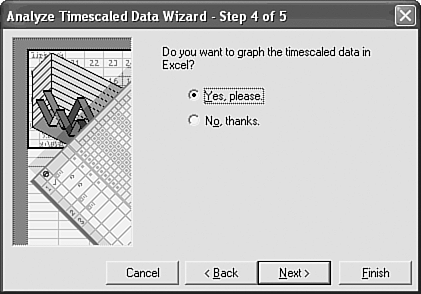5. In Step 5 of the wizard, you click Export Data if you're ready to export the data, you click Back if you want to change the parameters, or you click Cancel if you want to abort the wizard (see Figure 15.26). Click Export Data to finish the wizard.

##### Figure 15.26. The final step of the wizard gives you the option to back up, continue, or cancel.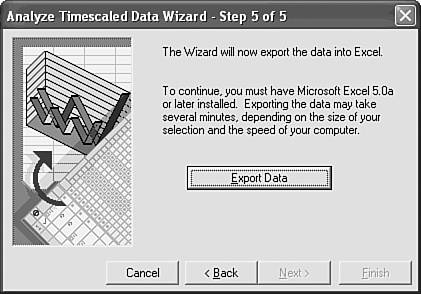When Project exports the data, Microsoft Excel automatically opens, and the exported data is displayed in a graph if you elected to create one; otherwise , the data is displayed in a worksheet. The default graph that's created by Excel is three-dimensional (see Figure 15.27). If you chose the default date range, the graph has many zero-valued points plotted for values after the status date.

##### Figure 15.27. The initial graph is three-dimensional, and it also includes many zero-valued data points.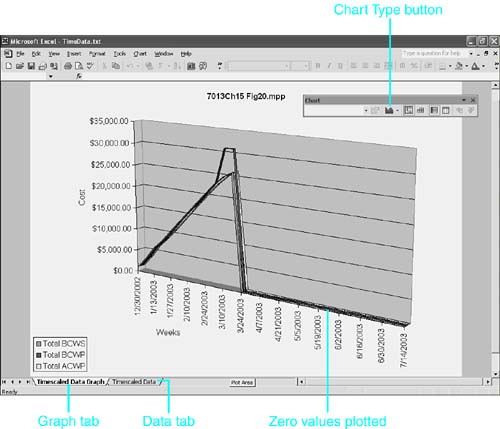6. Change the chart to a line chart by clicking the Chart Type button on the Chart toolbar and selecting Line Chart.

7. Edit the data on the Timescaled Data tab to remove the points that were plotted for zeros.

Click the Timescaled Data tab. The data that's actually plotted in the graph is at the bottom of this worksheet, where Excel has calculated the sums of the timescaled values for all tasks. Press Ctrl+End to jump to the last time period in the last row, and then press Home to jump to Column A for that row.

The labels that are used in the graph legend are in Column B, but those labels are also used in the SUMIF() formulas in the cells to the right. You should not edit the labels unless you first paste the formula values over the formulas. To replace the formulas with values, select all the formulas in the last three rows and press Ctrl+C (or choose Edit, Copy). Then choose Edit, Paste Special, choose Paste Values, and click OK. You can then change the labels to Planned Value, Earned Value, and Actual Costs.

While you have the values selected, change the display to zero decimal places, to reduce some of the clutter in your graph. Choose Format, Cells, click the Number tab, and change Decimal Places to 0.

Finally, delete the zero-valued cells in the last three rows that occur after the status date. Deleting them keeps Excel from plotting those cells in the graph.

Figure 15.28 shows the graph after it is changed to a line graph, after the zero values are removed and the other numbers are rounded to whole numbers, and after the legend text is changed as described earlier.

##### Figure 15.28. The graph of the core earned value calculations illustrates changes over time in the performance of the earned value and the actual costs relative to the planned value.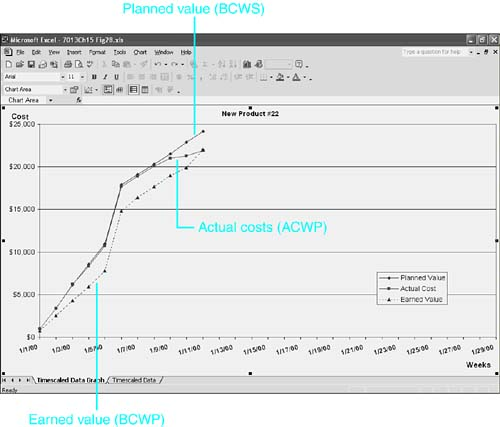8. Excel always gives the file it creates for the exported data the name TimeData.txt . Save the file as an Excel file with a new name, in order to preserve the graph.

What can you tell from a graph of earned value measurements such as Figure 15.28? First, for any status date along the X-axis, the vertical distance between the Earned Value curve and the Planned Value curve measures the Schedule Variance (SV). In this example, Planned Value is above Earned Value, which means that SV is negative at the current status date (the last plotted points). That's unfavorable because it means that at the present performance level, the project won't be completed on time. The vertical distance between the Earned Value curve and the Actual Costs curve at any date measures the Cost Variance (CV) as of that date. In this case, that is close to zero as of the current status date, although it's been negative for most of the project because Actual Cost has been higher than Earned Value. When CV is negative, it means that you are paying more than you budgeted for the amount of value you're actually generating. If the project continues until all work is completed (which the unfavorable SV predicts will be past the scheduled finish date), that work will have cost more than budgeted.

An earned value table can tell you just as much as the graph (or maybe more) for any chosen status date. The graph adds the ability to see the trend in the variancesto see whether things have been getting better or worse . In this example, the SV continues to be unfavorable, which means that you have not resolved the problem(s) that cause you to be behind schedule. But the CV has recently been reduced to zero, and that means that you have begun to bring costs down to the level they were scheduled for the amount of work that is actually being done.

If the purpose of earned value analysis is to tell you the type of corrective measures you need to take to meet your project goals, the graph helps you assess whether your corrective measures are working.

 < Day Day Up >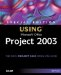Special Edition Using Microsoft Office Project 2003
ISBN: 0789730723
EAN: 2147483647
Year: 2004
Pages: 283
Authors: Tim Pyron

Similar book on Amazon